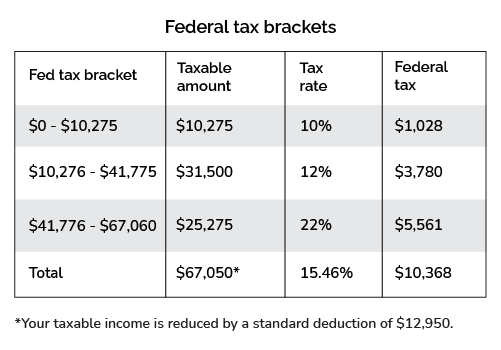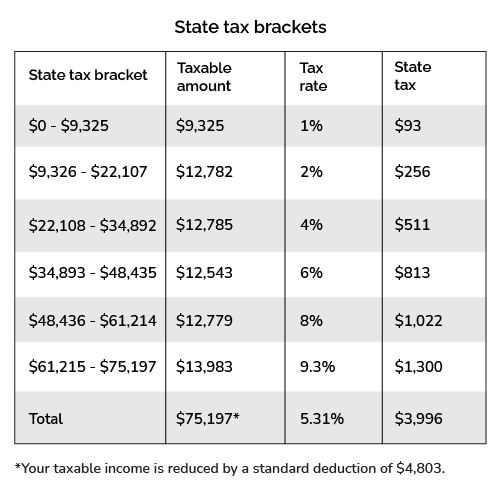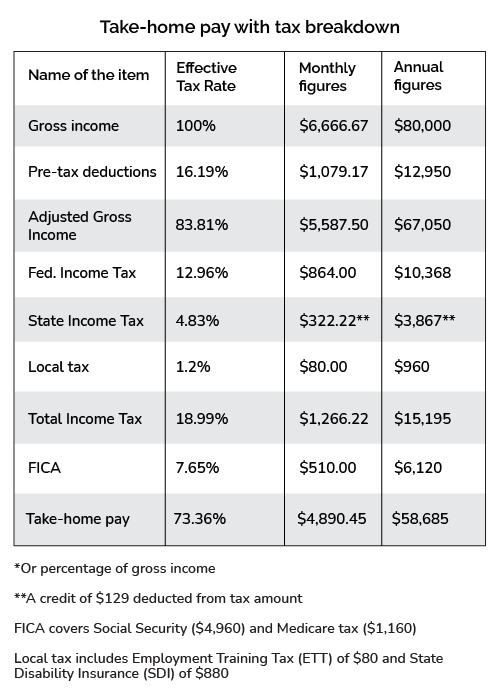# California Tax Calculator

Created by Tibor Pál, PhD candidate and Arturo Barrantes
Reviewed by Dominik Czernia, PhD and Steven Wooding
Based on research by
Tax Foundation;
Last updated: Jun 05, 2023

Our helpful California tax calculator (which you can use as a paycheck calculator for California or a California payroll calculator) takes the hassle out of figuring out how much of your adjusted gross income (AGI) you will put toward taxes this year.

In this article, we cover everything from how to calculate income tax in California state, tax brackets, state taxes, deductions, and effective tax rates, even including every case for your filing status.

Although challenging, do not worry! We promise you will end up being able to calculate the California income tax rate by yourself.

## Which are the California taxes?

The taxes a Californian citizen has to pay include the state income tax, the local income tax, and the general ones: the FICA tax and the federal income tax.

Tax type / Filing status

Single

Married & Jointly

Married & Separately

FICA

7.65%: Social security (6.2%) + medicare (1.45%) taxes

Federal income tax

Progressive tax brackets from 10% to 37%

Deductions in USD

12,950

25,900

12,950

19,400

State income tax

Progressive tax brackets from 1% to 12.3%

Local tax

Only San Francisco has a local tax: 0.38% paid by the employer (payroll tax).

Sales tax

7.25% - 10.75%: Tax paid for the sale of certain goods and services.

The California tax calculator already includes all the specifics indicated above; however, since we want to make everything crystal clear, we will include the next table: tax brackets for the California state income tax. You should check the tax bracket calculator in case you need more information about the federal income tax.

Single filers California tax brackets 2022. It also applies to married/registered domestic partners who fill separately.

Tax rate

Taxable income bracket

Tax owed

Standard deduction

Personal exemption

1%

$0 to$9,325

1% of taxable income

$4803 Standard deduction or personal exemption is structured as a tax credit.$129 credit

2%

$9,326 to$22,107

$93.25 + 2% of the amount over$9,325

4%

$22,108 to$34,892

$348.87 + 4% of the amount over$22,108

6%

$34,893 to$48,435

$860.23 + 6% of the amount over$34,893

8%

$48,436 to$61,214

$1672.75 + 8% of the amount over$48,436

9.3%

$61,214 to$312,686

$2694.99 + 9.3% of the amount over$61,214

10.3%

$312,687 to$375,221

$26,081.79 + 10.3% of the amount over$312,687

11.3%

$375,222 to$625,369

$32,522.79 + 11.3% of the amount over$375,222

12.3%

$625,370 to$1,000,000

$60,789.40 + 12.3% over$625,370

13.3%

$1,000,001 or more$106,868.89 + 13.3% over $1,000,001 It is important to add that for single taxpayers, there is a credit phase-out of$6 for each $2,500 of federal AGI above$212,288. In any case, California state tax credit cannot be reduced below zero.

Married filing jointly California tax brackets 2022. It also applies to registered domestic filing jointly and qualified widow(er).

Tax rate

Taxable income bracket

Tax owed

Standard deduction

Personal exemption

1%

$0 to$18,650

1% of taxable income

$9606 Standard deduction or personal exemption is structured as a tax credit.$258 credit

2%

$18,651 to$44,214

$186.50 + 2% of the amount over$18,650

4%

$44,215 to$69,784

$697.76 + 4% of the amount over$44,214

6%

$69,785 to$96,870

$1,720.52 + 6% of the amount over$69,784

8%

$96,871 to$122,428

$3,345.62 + 8% of the amount over$96,870

9.3%

$122,429 to$625,372

$53,90.18 + 9.3% of the amount over$122,428

10.3%

$625,373 to$750,442

$52,163.88 + 10.3% of the amount over$625,373

11.3%

$750,443 to$1,000,000

$65,046 + 11.3% of the amount over$750,442

12.3%

$1,000,001 to$1,250,738

$93,245.93 + 12.3% of the amount over$1,000,000

13.3%

$1,250,739 or more$124,086.58 + 13.3% of the amount over $1,250,739 ## How do I calculate California income tax? Before we start, we need a set of information, including your filing status, as indicated in the California income tax rate table we shared above. Then: 1. Suppose you are a single filer with an AGI of$80,000 living in California state and want to know your 2022 total income tax. Let's start with your FICA, which will be: $\small 80,\!000 \times 7.65 \%$.

2. Now, it is time for your federal income tax. After considering the 2022 deductions we showed above, your taxable income is $67,050 (that is because $\small 80,\!000 - 12,\!950 = 67,\!050$). Consequently, considering the federal tax progressive income, you pay$4,807.50 plus 22% of the amount over $41,775, which equals a total federal tax of$10,368.

Let's explain that a bit more: try the California state tax calculator and see the second table. You will notice that you reach the 22% bracket, but your income is only partially taxed at that percentage. Check the following table:1. We continue calculating the California state income tax. Here you could go ahead and check the third table: State tax brackets, which will be shown at the end of this section.

To learn how to calculate it, follow the next steps:

• Subtract the deductions and exemptions. That means we have an AGI of $\small 80,\!000 - 4803$, accordingly to the tables above. Consequently, we get $75,197 of taxable income. • According to the bottom table, since we are in the$61,215 to $312,686 bracket, we must pay$2,695 plus 9.3% of the amount over $61,214 as taxes. In other words, $\small 2695 + (75,\!197-61,\!215)\times 9.3 \%$ which equals$3,996. See the following table as a summary:We still have not considered the $129 personal exemption. However, we will consider it for calculating your take-home pay. Consequently, the California state income tax becomes$3867.

1. We need to consider the local tax in the California tax calculator 2022: Local tax includes Employment Training Tax (ETT) and State Disability Insurance (SDI). The ETT rate for 2022 is 0.1%, while the SDI rate is 1.1%, making the ETT tax and the SDI tax a combined value of $960, which is the local income tax. 2. Finally, we subtract all the taxes: $\small 80,\!000\! -\! 10,\!368\! -\! 3,\!867\! -\! 960\! -\! 6,\!120$. The take-home pay amount is$58,685, as you can see in the California state tax calculator.In addition, you can estimate your take-home pay for a selected pay frequency – therefore using our tool as a California payroll calculator or payroll calculator for California. For example, if you want to see your monthly take-home pay, you need to select monthly pay frequency, resulting in estimated monthly take-home pay of $4,890.45. ## How to calculate California state income tax for a married couple? You can use this Omni Calculator tool paycheck calculator for California or do as follows: 1. Suppose you are married, filing jointly, and making the median income in California state:$107,000 annually. Subtract 7.65% of the adjusted gross income (AGI). That will go to your FICA: $8,186. 2. Find your marginal tax rate. After deductions ($25,900), we have a marginal tax rate of 12% on $107,000 -$25,900 = $81,100, but remember, the marginal tax rate is only the last bracket we fit in. In this case, you will be taxed progressively: 10% up to$20,550, equivalent to $2055, and the rest at 12%. Thus, we must pay$2,055 plus 12% of the amount over $20,550 (the last bracket), or$9,321 in total.

3. Obtain the California paycheck tax: Because of your AGI, your standard deduction is $9,606 and a couple-exemption of$258 credit tax. Hence, your taxable income becomes $97,394. The state income tax is$1,625 plus 8% of the amount over $96,871, which equals$3387.54 or $3388. 4. To get the final state tax, we need to subtract the tax credit ($258). As you can confirm in the California state tax calculator, the result becomes $3,130. 5. Regarding the local income tax, we have to consider ETT and SDI (0.1%, and 1.1%, respectively). Both makes: $1,177 + $118 =$1,284

### How do I calculate the property tax in California?

You can use the California property tax calculator or do as follows:

1. Get your assessed home value. Do not forget to request your $7,000 deduction if it is your first house. 2. Find the property tax rate for your house location. For example, Alameda county has a property tax rate of 0.78% in 2022. 3. Multiply your assessed home value by the property tax rate. And that's it. You are ready to go! ### How much tax do I owe if I earn$34,000 in California?

The estimated total annual tax is $5,833. You can calculate it as follows: 1. Subtract the pre-tax deduction:$12,950. Based on your adjusted gross income, $21,050, calculate your federal tax rate: 12%. Remember, it is a progressive tax. The tax to pay is$2,321.

2. Subtract the state tax deduction: $4,803. Similarly to the above, the tax to pay is$503 (already minus tax $129 credit). 3. Determine local taxes. It includes ETT (1.1%) and SDI (0.01%). The result is$408.

4. Calculate FICA tax (7.65% of AGI). It is $2,601. 5. Sum all taxes above: The total annual tax is$5,833.

## Disclaimer

You should consider the California tax calculator as a model for financial approximation. All figures and balances are estimates based on the data you provided in the specifications that are not exhaustive, despite our best effort. In addition, the calculator potentially omits information relevant to determining the exact income tax figures.

For these reasons, we created the California tax calculator for instructional purposes only. Still, if you experience a relevant drawback or encounter any inaccuracy, we are always pleased to receive helpful feedback and advice.

Tibor Pál, PhD candidate and Arturo Barrantes
Payroll taxes
$/year Pay frequency Daily Year of income 2022 What is your filling status? Single Property tax Assessed home value$
Location
Alameda County
Applied property tax rate
%
Results
Display more details
summary table only
People also viewed…

### After-tax cost of debt

Our after-tax cost of debt calculator allows you to calculate the required return of a company's debt in no time.

### Circumference

Use this free circumference calculator to find the area, circumference and diameter of a circle.

### Meat footprint

Check out the impact meat has on the environment and your health.

### Present value of growth opportunities (PVGO)

Discover the potential of company growth with our PVGO calculator - understand company valuation better and invest smartly!